# Shape Constraints for Ethics with Tensorflow Lattice

## Overview

This tutorial demonstrates how the TensorFlow Lattice (TFL) library can be used to train models that behave responsibly, and do not violate certain assumptions that are ethical or fair. In particular, we will focus on using monotonicity constraints to avoid unfair penalization of certain attributes. This tutorial includes demonstrations of the experiments from the paper Deontological Ethics By Monotonicity Shape Constraints by Serena Wang and Maya Gupta, published at AISTATS 2020.

We will use TFL canned estimators on public datasets, but note that everything in this tutorial can also be done with models constructed from TFL Keras layers.

Before proceeding, make sure your runtime has all required packages installed (as imported in the code cells below).

## Setup

Installing TF Lattice package:

````pip install tensorflow-lattice tensorflow_decision_forests seaborn`
```

Importing required packages:

``````import tensorflow as tf
import tensorflow_lattice as tfl
import tensorflow_decision_forests as tfdf

import logging
import matplotlib.pyplot as plt
import numpy as np
import os
import pandas as pd
import seaborn as sns
from sklearn.model_selection import train_test_split
import sys
import tempfile
logging.disable(sys.maxsize)
``````
```WARNING:root:TF Parameter Server distributed training not available (this is expected for the pre-build release).
```

Default values used in this tutorial:

``````# List of learning rate hyperparameters to try.
# For a longer list of reasonable hyperparameters, try [0.001, 0.01, 0.1].
LEARNING_RATES = [0.01]
# Default number of training epochs and batch sizes.
NUM_EPOCHS = 1000
BATCH_SIZE = 1000
# Directory containing dataset files.
DATA_DIR = 'https://raw.githubusercontent.com/serenalwang/shape_constraints_for_ethics/master'
``````

# Case study #1: Law school admissions

In the first part of this tutorial, we will consider a case study using the Law School Admissions dataset from the Law School Admissions Council (LSAC). We will train a classifier to predict whether or not a student will pass the bar using two features: the student's LSAT score and undergraduate GPA.

Suppose that the classifier’s score was used to guide law school admissions or scholarships. According to merit-based social norms, we would expect that students with higher GPA and higher LSAT score should receive a higher score from the classifier. However, we will observe that it is easy for models to violate these intuitive norms, and sometimes penalize people for having a higher GPA or LSAT score.

To address this unfair penalization problem, we can impose monotonicity constraints so that a model never penalizes higher GPA or higher LSAT score, all else equal. In this tutorial, we will show how to impose those monotonicity constraints using TFL.

``````# Load data file.
law_file_name = 'lsac.csv'
law_file_path = os.path.join(DATA_DIR, law_file_name)
``````

Preprocess dataset:

``````# Define label column name.
LAW_LABEL = 'pass_bar'
``````
``````def preprocess_law_data(input_df):
# Drop rows with where the label or features of interest are missing.
output_df = input_df[~input_df[LAW_LABEL].isna() & ~input_df['ugpa'].isna() &
(input_df['ugpa'] > 0) & ~input_df['lsat'].isna()]
return output_df

law_df = preprocess_law_data(raw_law_df)
``````

### Split data into train/validation/test sets

``````def split_dataset(input_df, random_state=888):
"""Splits an input dataset into train, val, and test sets."""
train_df, test_val_df = train_test_split(
input_df, test_size=0.3, random_state=random_state)
val_df, test_df = train_test_split(
test_val_df, test_size=0.66, random_state=random_state)
return train_df, val_df, test_df

law_train_df, law_val_df, law_test_df = split_dataset(law_df)
``````

### Visualize data distribution

First we will visualize the distribution of the data. We will plot the GPA and LSAT scores for all students that passed the bar and also for all students that did not pass the bar.

``````def plot_dataset_contour(input_df, title):
plt.rcParams['font.family'] = ['serif']
g = sns.jointplot(
x='ugpa',
y='lsat',
data=input_df,
kind='kde',
xlim=[1.4, 4],
ylim=[0, 50])
g.plot_joint(plt.scatter, c='b', s=10, linewidth=1, marker='+')
g.ax_joint.collections.set_alpha(0)
g.fig.suptitle(title, fontsize=14)
# Adust plot so that the title fits.
plt.show()
``````
``````law_df_pos = law_df[law_df[LAW_LABEL] == 1]
plot_dataset_contour(
law_df_pos, title='Distribution of students that passed the bar')
``````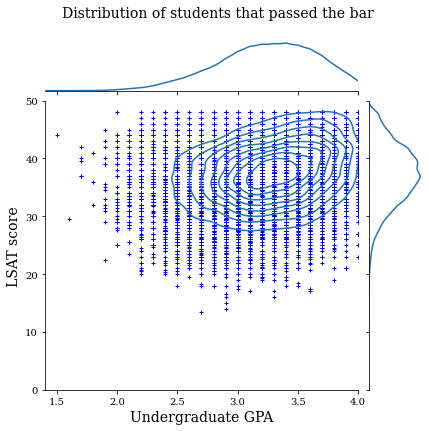``````law_df_neg = law_df[law_df[LAW_LABEL] == 0]
plot_dataset_contour(
law_df_neg, title='Distribution of students that failed the bar')
``````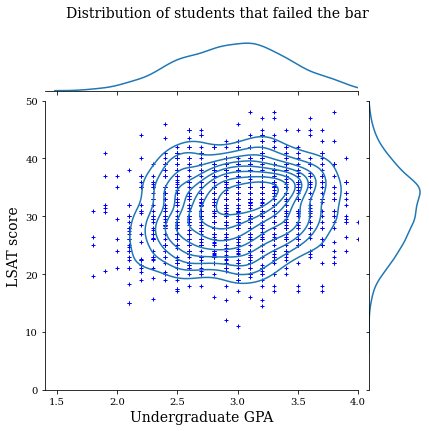## Train calibrated linear model to predict bar exam passage

Next, we will train a calibrated linear model from TFL to predict whether or not a student will pass the bar. The two input features will be LSAT score and undergraduate GPA, and the training label will be whether the student passed the bar.

We will first train a calibrated linear model without any constraints. Then, we will train a calibrated linear model with monotonicity constraints and observe the difference in the model output and accuracy.

### Helper functions for training a TFL calibrated linear estimator

These functions will be used for this law school case study, as well as the credit default case study below.

``````def train_tfl_estimator(train_df, monotonicity, learning_rate, num_epochs,
batch_size, get_input_fn,
get_feature_columns_and_configs):
"""Trains a TFL calibrated linear estimator.

Args:
train_df: pandas dataframe containing training data.
monotonicity: if 0, then no monotonicity constraints. If 1, then all
features are constrained to be monotonically increasing.
num_epochs: number of training epochs.
batch_size: batch size for each epoch. None means the batch size is the full
dataset size.
get_input_fn: function that returns the input_fn for a TF estimator.
get_feature_columns_and_configs: function that returns TFL feature columns
and configs.

Returns:
estimator: a trained TFL calibrated linear estimator.

"""
feature_columns, feature_configs = get_feature_columns_and_configs(
monotonicity)

model_config = tfl.configs.CalibratedLinearConfig(
feature_configs=feature_configs, use_bias=False)

estimator = tfl.estimators.CannedClassifier(
feature_columns=feature_columns,
model_config=model_config,
feature_analysis_input_fn=get_input_fn(input_df=train_df, num_epochs=1),

estimator.train(
input_fn=get_input_fn(
input_df=train_df, num_epochs=num_epochs, batch_size=batch_size))
return estimator

def optimize_learning_rates(
train_df,
val_df,
test_df,
monotonicity,
learning_rates,
num_epochs,
batch_size,
get_input_fn,
get_feature_columns_and_configs,
):
"""Optimizes learning rates for TFL estimators.

Args:
train_df: pandas dataframe containing training data.
val_df: pandas dataframe containing validation data.
test_df: pandas dataframe containing test data.
monotonicity: if 0, then no monotonicity constraints. If 1, then all
features are constrained to be monotonically increasing.
learning_rates: list of learning rates to try.
num_epochs: number of training epochs.
batch_size: batch size for each epoch. None means the batch size is the full
dataset size.
get_input_fn: function that returns the input_fn for a TF estimator.
get_feature_columns_and_configs: function that returns TFL feature columns
and configs.

Returns:
A single TFL estimator that achieved the best validation accuracy.
"""
estimators = []
train_accuracies = []
val_accuracies = []
test_accuracies = []
for lr in learning_rates:
estimator = train_tfl_estimator(
train_df=train_df,
monotonicity=monotonicity,
learning_rate=lr,
num_epochs=num_epochs,
batch_size=batch_size,
get_input_fn=get_input_fn,
get_feature_columns_and_configs=get_feature_columns_and_configs)
estimators.append(estimator)
train_acc = estimator.evaluate(
input_fn=get_input_fn(train_df, num_epochs=1))['accuracy']
val_acc = estimator.evaluate(
input_fn=get_input_fn(val_df, num_epochs=1))['accuracy']
test_acc = estimator.evaluate(
input_fn=get_input_fn(test_df, num_epochs=1))['accuracy']
print('accuracies for learning rate %f: train: %f, val: %f, test: %f' %
(lr, train_acc, val_acc, test_acc))
train_accuracies.append(train_acc)
val_accuracies.append(val_acc)
test_accuracies.append(test_acc)
max_index = val_accuracies.index(max(val_accuracies))
return estimators[max_index]
``````

### Helper functions for configuring law school dataset features

These helper functions are specific to the law school case study.

``````def get_input_fn_law(input_df, num_epochs, batch_size=None):
"""Gets TF input_fn for law school models."""
return tf.compat.v1.estimator.inputs.pandas_input_fn(
x=input_df[['ugpa', 'lsat']],
y=input_df['pass_bar'],
num_epochs=num_epochs,
batch_size=batch_size or len(input_df),
shuffle=False)

def get_feature_columns_and_configs_law(monotonicity):
"""Gets TFL feature configs for law school models."""
feature_columns = [
tf.feature_column.numeric_column('ugpa'),
tf.feature_column.numeric_column('lsat'),
]
feature_configs = [
tfl.configs.FeatureConfig(
name='ugpa',
lattice_size=2,
pwl_calibration_num_keypoints=20,
monotonicity=monotonicity,
pwl_calibration_always_monotonic=False),
tfl.configs.FeatureConfig(
name='lsat',
lattice_size=2,
pwl_calibration_num_keypoints=20,
monotonicity=monotonicity,
pwl_calibration_always_monotonic=False),
]
return feature_columns, feature_configs
``````

### Helper functions for visualization of trained model outputs

``````def get_predicted_probabilities(estimator, input_df, get_input_fn):
if isinstance(estimator, tf.estimator.Estimator):
predictions = estimator.predict(
input_fn=get_input_fn(input_df=input_df, num_epochs=1))
return [prediction['probabilities'] for prediction in predictions]
else:
return estimator.predict(tfdf.keras.pd_dataframe_to_tf_dataset(input_df))

def plot_model_contour(estimator, input_df, num_keypoints=20):
x = np.linspace(min(input_df['ugpa']), max(input_df['ugpa']), num_keypoints)
y = np.linspace(min(input_df['lsat']), max(input_df['lsat']), num_keypoints)

x_grid, y_grid = np.meshgrid(x, y)

positions = np.vstack([x_grid.ravel(), y_grid.ravel()])
plot_df = pd.DataFrame(positions.T, columns=['ugpa', 'lsat'])
plot_df[LAW_LABEL] = np.ones(len(plot_df))
predictions = get_predicted_probabilities(
estimator=estimator, input_df=plot_df, get_input_fn=get_input_fn_law)
grid_predictions = np.reshape(predictions, x_grid.shape)

plt.rcParams['font.family'] = ['serif']
plt.contour(
x_grid,
y_grid,
grid_predictions,
colors=('k',),
levels=np.linspace(0, 1, 11))
plt.contourf(
x_grid,
y_grid,
grid_predictions,
cmap=plt.cm.bone,
levels=np.linspace(0, 1, 11))  # levels=np.linspace(0,1,8));
plt.xticks(fontsize=20)
plt.yticks(fontsize=20)

cbar = plt.colorbar()
cbar.ax.set_ylabel('Model score', fontsize=20)
cbar.ax.tick_params(labelsize=20)

plt.ylabel('LSAT score', fontsize=20)
``````

## Train unconstrained (non-monotonic) calibrated linear model

``````nomon_linear_estimator = optimize_learning_rates(
train_df=law_train_df,
val_df=law_val_df,
test_df=law_test_df,
monotonicity=0,
learning_rates=LEARNING_RATES,
batch_size=BATCH_SIZE,
num_epochs=NUM_EPOCHS,
get_input_fn=get_input_fn_law,
get_feature_columns_and_configs=get_feature_columns_and_configs_law)
``````
```2022-04-19 11:30:46.377009: E tensorflow/stream_executor/cuda/cuda_driver.cc:271] failed call to cuInit: CUDA_ERROR_NO_DEVICE: no CUDA-capable device is detected
accuracies for learning rate 0.010000: train: 0.949061, val: 0.945876, test: 0.951781
```
``````plot_model_contour(nomon_linear_estimator, input_df=law_df)
``````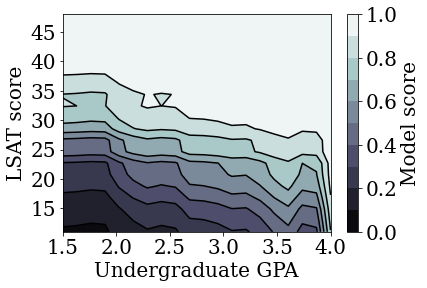## Train monotonic calibrated linear model

``````mon_linear_estimator = optimize_learning_rates(
train_df=law_train_df,
val_df=law_val_df,
test_df=law_test_df,
monotonicity=1,
learning_rates=LEARNING_RATES,
batch_size=BATCH_SIZE,
num_epochs=NUM_EPOCHS,
get_input_fn=get_input_fn_law,
get_feature_columns_and_configs=get_feature_columns_and_configs_law)
``````
```accuracies for learning rate 0.010000: train: 0.949249, val: 0.945447, test: 0.951781
```
``````plot_model_contour(mon_linear_estimator, input_df=law_df)
``````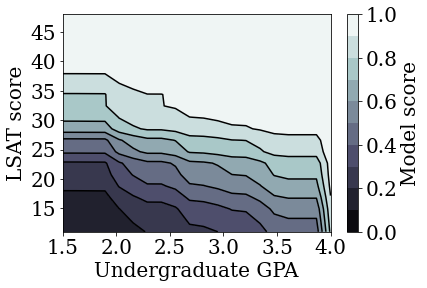## Train other unconstrained models

We demonstrated that TFL calibrated linear models could be trained to be monotonic in both LSAT score and GPA without too big of a sacrifice in accuracy.

But, how does the calibrated linear model compare to other types of models, like deep neural networks (DNNs) or gradient boosted trees (GBTs)? Do DNNs and GBTs appear to have reasonably fair outputs? To address this question, we will next train an unconstrained DNN and GBT. In fact, we will observe that the DNN and GBT both easily violate monotonicity in LSAT score and undergraduate GPA.

### Train an unconstrained Deep Neural Network (DNN) model

The architecture was previously optimized to achieve high validation accuracy.

``````feature_names = ['ugpa', 'lsat']

dnn_estimator = tf.estimator.DNNClassifier(
feature_columns=[
tf.feature_column.numeric_column(feature) for feature in feature_names
],
hidden_units=[100, 100],
activation_fn=tf.nn.relu)

dnn_estimator.train(
input_fn=get_input_fn_law(
law_train_df, batch_size=BATCH_SIZE, num_epochs=NUM_EPOCHS))
dnn_train_acc = dnn_estimator.evaluate(
input_fn=get_input_fn_law(law_train_df, num_epochs=1))['accuracy']
dnn_val_acc = dnn_estimator.evaluate(
input_fn=get_input_fn_law(law_val_df, num_epochs=1))['accuracy']
dnn_test_acc = dnn_estimator.evaluate(
input_fn=get_input_fn_law(law_test_df, num_epochs=1))['accuracy']
print('accuracies for DNN: train: %f, val: %f, test: %f' %
(dnn_train_acc, dnn_val_acc, dnn_test_acc))
``````
```accuracies for DNN: train: 0.949374, val: 0.948024, test: 0.951338
```
``````plot_model_contour(dnn_estimator, input_df=law_df)
``````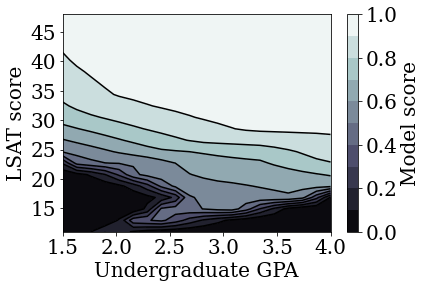### Train an unconstrained Gradient Boosted Trees (GBT) model

The tree structure was previously optimized to achieve high validation accuracy.

``````law_train_ds = tfdf.keras.pd_dataframe_to_tf_dataset(
law_train_df, label='pass_bar')
law_test_ds = tfdf.keras.pd_dataframe_to_tf_dataset(
law_test_df, label='pass_bar')
law_val_ds = tfdf.keras.pd_dataframe_to_tf_dataset(law_val_df, label='pass_bar')

features=[tfdf.keras.FeatureUsage(name=name) for name in feature_names],
exclude_non_specified_features=True,
num_trees=20,
max_depth=4,
growing_strategy='BEST_FIRST_GLOBAL',
random_seed=42,
temp_directory=tempfile.mkdtemp(),
)
tree_model.compile(metrics=[tf.keras.metrics.BinaryAccuracy(name='accuracy')])
tree_model.fit(law_train_ds, validation_data=law_val_ds, verbose=0)

tree_train_acc = tree_model.evaluate(law_train_ds, verbose=0)
tree_val_acc = tree_model.evaluate(law_val_ds, verbose=0)
tree_test_acc = tree_model.evaluate(law_test_ds, verbose=0)
print('accuracies for GBT: train: %f, val: %f, test: %f' %
(tree_train_acc, tree_val_acc, tree_test_acc))
``````
```/tmpfs/src/tf_docs_env/lib/python3.7/site-packages/tensorflow_decision_forests/keras/core.py:2036: FutureWarning: In a future version of pandas all arguments of DataFrame.drop except for the argument 'labels' will be keyword-only
features_dataframe = dataframe.drop(label, 1)
[INFO kernel.cc:1001] Use fast generic engine
WARNING: AutoGraph could not transform <function simple_ml_inference_op_with_handle at 0x7fdefa48f7a0> and will run it as-is.
Please report this to the TensorFlow team. When filing the bug, set the verbosity to 10 (on Linux, `export AUTOGRAPH_VERBOSITY=10`) and attach the full output.
Cause: could not get source code
To silence this warning, decorate the function with @tf.autograph.experimental.do_not_convert
accuracies for GBT: train: 0.949625, val: 0.948024, test: 0.951559
```
``````plot_model_contour(tree_model, input_df=law_df)
``````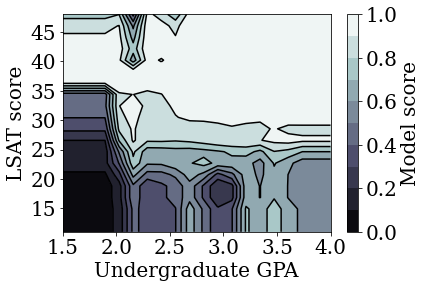# Case study #2: Credit Default

The second case study that we will consider in this tutorial is predicting an individual's credit default probability. We will use the Default of Credit Card Clients dataset from the UCI repository. This data was collected from 30,000 Taiwanese credit card users and contains a binary label of whether or not a user defaulted on a payment in a time window. Features include marital status, gender, education, and how long a user is behind on payment of their existing bills, for each of the months of April-September 2005.

As we did with the first case study, we again illustrate using monotonicity constraints to avoid unfair penalization: if the model were to be used to determine a user’s credit score, it could feel unfair to many if they were penalized for paying their bills sooner, all else equal. Thus, we apply a monotonicity constraint that keeps the model from penalizing early payments.

``````# Load data file.
credit_file_name = 'credit_default.csv'
credit_file_path = os.path.join(DATA_DIR, credit_file_name)
``````
``````# Define label column name.
CREDIT_LABEL = 'default'
``````

### Split data into train/validation/test sets

``````credit_train_df, credit_val_df, credit_test_df = split_dataset(credit_df)
``````

### Visualize data distribution

First we will visualize the distribution of the data. We will plot the mean and standard error of the observed default rate for people with different marital statuses and repayment statuses. The repayment status represents the number of months a person is behind on paying back their loan (as of April 2005).

``````def get_agg_data(df, x_col, y_col, bins=11):
xbins = pd.cut(df[x_col], bins=bins)
data = df[[x_col, y_col]].groupby(xbins).agg(['mean', 'sem'])
return data

def plot_2d_means_credit(input_df, x_col, y_col, x_label, y_label):
plt.rcParams['font.family'] = ['serif']
_, ax = plt.subplots(nrows=1, ncols=1)
plt.setp(ax.spines.values(), color='black', linewidth=1)
ax.tick_params(
direction='in', length=6, width=1, top=False, right=False, labelsize=18)
df_single = get_agg_data(input_df[input_df['MARRIAGE'] == 1], x_col, y_col)
df_married = get_agg_data(input_df[input_df['MARRIAGE'] == 2], x_col, y_col)
ax.errorbar(
df_single[(x_col, 'mean')],
df_single[(y_col, 'mean')],
xerr=df_single[(x_col, 'sem')],
yerr=df_single[(y_col, 'sem')],
color='orange',
marker='s',
capsize=3,
capthick=1,
label='Single',
markersize=10,
linestyle='')
ax.errorbar(
df_married[(x_col, 'mean')],
df_married[(y_col, 'mean')],
xerr=df_married[(x_col, 'sem')],
yerr=df_married[(y_col, 'sem')],
color='b',
marker='^',
capsize=3,
capthick=1,
label='Married',
markersize=10,
linestyle='')
leg = ax.legend(loc='upper left', fontsize=18, frameon=True, numpoints=1)
ax.set_xlabel(x_label, fontsize=18)
ax.set_ylabel(y_label, fontsize=18)
ax.set_ylim(0, 1.1)
ax.set_xlim(-2, 8.5)
ax.patch.set_facecolor('white')
leg.get_frame().set_edgecolor('black')
leg.get_frame().set_facecolor('white')
leg.get_frame().set_linewidth(1)
plt.show()
``````
``````plot_2d_means_credit(credit_train_df, 'PAY_0', 'default',
'Repayment Status (April)', 'Observed default rate')
``````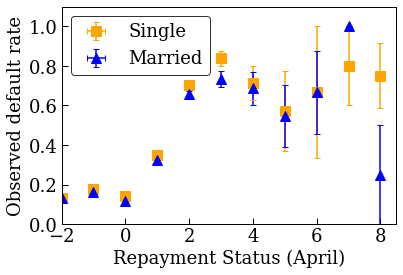## Train calibrated linear model to predict credit default rate

Next, we will train a calibrated linear model from TFL to predict whether or not a person will default on a loan. The two input features will be the person's marital status and how many months the person is behind on paying back their loans in April (repayment status). The training label will be whether or not the person defaulted on a loan.

We will first train a calibrated linear model without any constraints. Then, we will train a calibrated linear model with monotonicity constraints and observe the difference in the model output and accuracy.

### Helper functions for configuring credit default dataset features

These helper functions are specific to the credit default case study.

``````def get_input_fn_credit(input_df, num_epochs, batch_size=None):
"""Gets TF input_fn for credit default models."""
return tf.compat.v1.estimator.inputs.pandas_input_fn(
x=input_df[['MARRIAGE', 'PAY_0']],
y=input_df['default'],
num_epochs=num_epochs,
batch_size=batch_size or len(input_df),
shuffle=False)

def get_feature_columns_and_configs_credit(monotonicity):
"""Gets TFL feature configs for credit default models."""
feature_columns = [
tf.feature_column.numeric_column('MARRIAGE'),
tf.feature_column.numeric_column('PAY_0'),
]
feature_configs = [
tfl.configs.FeatureConfig(
name='MARRIAGE',
lattice_size=2,
pwl_calibration_num_keypoints=3,
monotonicity=monotonicity,
pwl_calibration_always_monotonic=False),
tfl.configs.FeatureConfig(
name='PAY_0',
lattice_size=2,
pwl_calibration_num_keypoints=10,
monotonicity=monotonicity,
pwl_calibration_always_monotonic=False),
]
return feature_columns, feature_configs
``````

### Helper functions for visualization of trained model outputs

``````def plot_predictions_credit(input_df,
estimator,
x_col,
x_label='Repayment Status (April)',
y_label='Predicted default probability'):
predictions = get_predicted_probabilities(
estimator=estimator, input_df=input_df, get_input_fn=get_input_fn_credit)
new_df = input_df.copy()
new_df.loc[:, 'predictions'] = predictions
plot_2d_means_credit(new_df, x_col, 'predictions', x_label, y_label)
``````

## Train unconstrained (non-monotonic) calibrated linear model

``````nomon_linear_estimator = optimize_learning_rates(
train_df=credit_train_df,
val_df=credit_val_df,
test_df=credit_test_df,
monotonicity=0,
learning_rates=LEARNING_RATES,
batch_size=BATCH_SIZE,
num_epochs=NUM_EPOCHS,
get_input_fn=get_input_fn_credit,
get_feature_columns_and_configs=get_feature_columns_and_configs_credit)
``````
```accuracies for learning rate 0.010000: train: 0.818762, val: 0.830065, test: 0.817172
```
``````plot_predictions_credit(credit_train_df, nomon_linear_estimator, 'PAY_0')
``````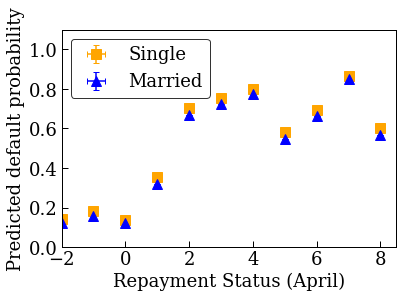## Train monotonic calibrated linear model

``````mon_linear_estimator = optimize_learning_rates(
train_df=credit_train_df,
val_df=credit_val_df,
test_df=credit_test_df,
monotonicity=1,
learning_rates=LEARNING_RATES,
batch_size=BATCH_SIZE,
num_epochs=NUM_EPOCHS,
get_input_fn=get_input_fn_credit,
get_feature_columns_and_configs=get_feature_columns_and_configs_credit)
``````
```accuracies for learning rate 0.010000: train: 0.818762, val: 0.830065, test: 0.817172
```
``````plot_predictions_credit(credit_train_df, mon_linear_estimator, 'PAY_0')
``````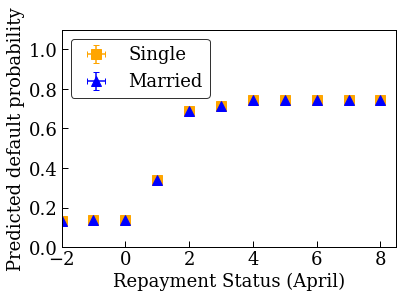[]
[]